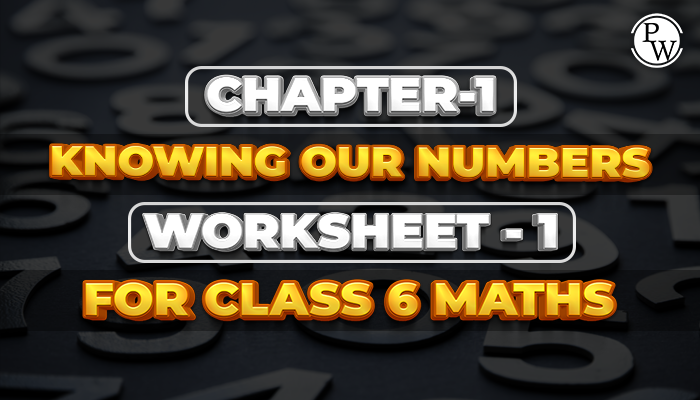# Chapter - 1 Knowing Our Numbers Worksheet - 1 for Class 6 Maths

#### Worksheets for Class 6 Maths

The Academic team of Physics Wallah prepared worksheets and questions for the Chapter- Knowing Our Numbers for all students who are in class 6. Physics Wallah questions for class 6 maths chapter Knowing Our Numbers consist of carefully selected numerical for class 6 maths and consist of step by step solutions of all questions with answers. Download the worksheet of the class 6 maths chapter Knowing Our Numbers and start solving the questions for reference using the solutions.Before solving the worksheet of Physics Wallah it is highly recommended to read the theory of the chapter and solve the questions given in your textbook. Students can also access the Class 6 Maths Notes from here.

## Solved Questions

Q1. 1 m = ______

1. 10 cm

2. 100 cm

3. 10 m

4. 1 km

Ans. Correct option is 2.

Q2. Write all possible 2-digit numbers using the digit 2,3 only once.

1. 23,32

2. 34,32

3. 23,43

4. 35,25

Ans. Correct Option is 1.

Q3. The product of 5 and 102 is

1. 128
2. 510
3. 256
4. 987

Ans. Correct option is 2.

Q4. Ram is 10 years old and Manu is 15 years old. Write the difference of their ages in roman system.

1. V
2. III
3. IV
4. VI

Ans. Ram’s age =10 years

Manu’s age =15 years , Then difference between their ages = 15 - 10 = 5 years

In roman = V

Q5. The successor of 99999 is

1. 100
2. 1000
3. 10,000
4. 1,00,000

Ans. The Successor of 99999 is = 99999 + 1 = 1,00,000

Q6. The value of (3+4+8-1+4-6) is

1. 13
2. 14
3. 10
4. 12

Ans. (3 + 4 + 8 - 1 + 4 - 6) = 3 + 4 + 8 + 4 - 1 - 6

= 19 - 7

= 12

Do follow NCERT Solutions for Class 6 Maths prepared by expert faculty of Physics Wallah.

Class 6 Maths Chapter - 1 Knowing Our Numbers download your free pdf copy from the below-mentioned link.